# Algebra 1 : How to find decimal equivalent to a percentage

## Example Questions

← Previous 1 3 4 5 6

### Example Question #1 : How To Find Decimal Equivalent To A Percentage

Which of the following numbers is the decimal equivalent of 80%?

0.8

0.08

0.008

8

80

0.8

Explanation:

80% can be thought of as 80% of one. Percents also refer to numbers out of 100, thus, our decimal can be calculated by taking 80/100. This would result in 8/10 after simplifying, which is equivalent to 0.8.

80% = 80/100 = 8/10 = "eight tenths," or 0.8

### Example Question #1 : How To Find Decimal Equivalent To A Percentage

Express 32% in decimal form.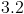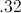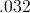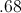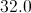Explanation:

To express a percentage as a decimal, imagine a decimal at the end of the percentage. Move the decimal over two places to the left, and you have the decimal expression of a percentage,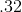### Example Question #1 : How To Find Decimal Equivalent To A Percentage

What is the simplest fraction that represents 88%?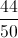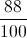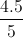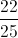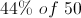Explanation:

The word percent, or per cent, means of every one hundred, so 88% can be expressed: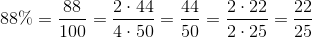### Example Question #2 : How To Find Decimal Equivalent To A Percentage

Andre borrows $80 from you. If he pays you back 40% of that money, how much money is he giving you? Possible Answers: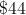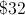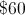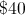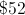Correct answer:Explanation: To find 40% of$80, you must turn 40% into a decimal.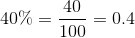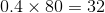### Example Question #1 : How To Find Decimal Equivalent To A Percentage

Convert 74% to a decimal.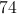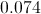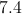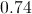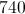Explanation:

The decimal in 74% is located between the 4 and the '%'. So think of 74% as 74.0%.  Then move that decimal point two digits to the left and erase the '%' so that it is written as 0.74

### Example Question #6 : How To Find Decimal Equivalent To A Percentage

Fill in the missing information.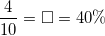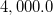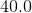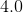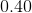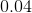Explanation:

When reading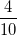aloud it readsout of, or "four tenths." This "four tenths" as a decimal is literally writtenWe could also use the other term given to find our answer. Decimals are found from percentages by moving the decimal point two places to the left. Given, the decimal moved results in### Example Question #7 : How To Find Decimal Equivalent To A Percentage

Fill in the missing information.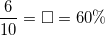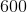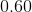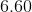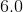Explanation:

When reading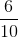aloud it readsout of, or "six tenths." This "six tenths" as a decimal is literally written.

We could also use the other term given to find our answer. Decimals are found from percentages by moving the decimal point two places to the left. Given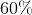, the decimal moved results in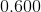or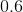### Example Question #8 : How To Find Decimal Equivalent To A Percentage

Solve the problem and convert the answer to a percentage.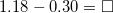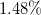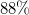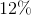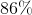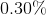Explanation: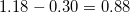. To convert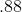to a percentage, the decimal is moved two places to the right, resulting in.

Alternatively, we would have converted the decimals to percetages from the beginnging.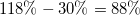, to do this we started with the decimal numbers given and moved the decimal point to the right two places.

### Example Question #9 : How To Find Decimal Equivalent To A Percentage

Convert this percentage to a decimal.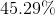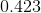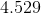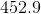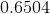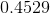Explanation:

Given the percentage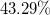, the decimal point must be moved two decimal places to the left, resulting in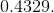This decimal can further be written as fraction,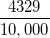which when reduced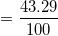.

Since percentages represent part of a whole, or part of 100,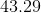is the final answer.

### Example Question #10 : How To Find Decimal Equivalent To A Percentage

Convert the answer to a decimal.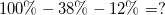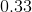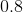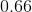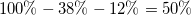.
Now to convert the percentage to a decimal all we do is move the decimal point two decimal places to the left. In this case, we began with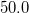. After moving the decimal point we end up with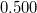as our result.